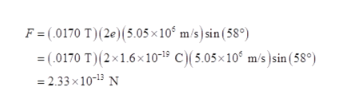# A beryllium-9 ion has a positive charge that is double the charge of a proton, and a mass of 1.50 ✕ 10−26 kg. At a particular instant, it is moving with a speed of 5.05 ✕ 106 m/s through a magnetic field. At this instant, its velocity makes an angle of 58° with the direction of the magnetic field at the ion's location. The magnitude of the field is 0.170 T. (a)What is the magnitude of the magnetic force (in N) on the ion? (b)What is the magnitude of the ion's acceleration (in m/s2) at this instant?

Question
10 views
A beryllium-9 ion has a positive charge that is double the charge of a proton, and a mass of 1.50 ✕ 10−26 kg. At a particular instant, it is moving with a speed of 5.05 ✕ 106 m/s through a magnetic field. At this instant, its velocity makes an angle of 58° with the direction of the magnetic field at the ion's location. The magnitude of the field is 0.170 T.

(a)
What is the magnitude of the magnetic force (in N) on the ion?

(b)
What is the magnitude of the ion's acceleration (in m/s2) at this instant?

check_circle

star
star
star
star
star
1 Rating
Step 1

(a) write the expression for magnetic force

Step 2

Substitute the valueshelp_outlineImage TranscriptioncloseF = (.0170 T)(2e) (5.05x 10 m/s) sin (58°) (0170 T)(2x16x101 C)(5.05x10° m/s)sin (58°) =2.33 x 1013 N fullscreen
Step 3

(b) Write the expression...

### Want to see the full answer?

See Solution

#### Want to see this answer and more?

Solutions are written by subject experts who are available 24/7. Questions are typically answered within 1 hour.*

See Solution
*Response times may vary by subject and question.
Tagged in

### Physics